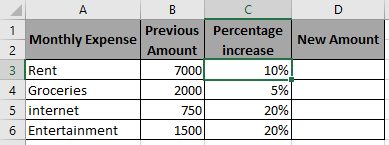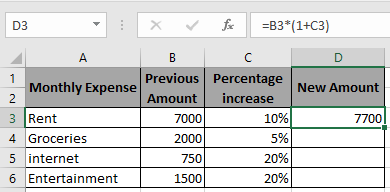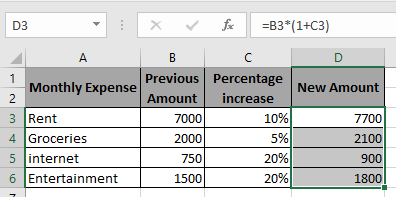How to Increase by percentage in Excel

In this article, we will learn about how to calculate the new or updated amount when the previous amount is increased by some percentage

If you need to find the new value when a previous value is increased by some percentage value. Use the below equation

New Amount = Previous Amount * (1 + Percentage Increase)

Let’s understand this operation using the example shown below

Here we will use the increased percentage value formula to get the new Amount for the data set.Use the Formula in D3 cell

=B3*(1+C3)As you can see in the above snapshot first New Amount after increment is 7700.

Copy the formula in the remaining cells to get the New Amount for the rest of the data.New Amount can be calculated using the above procedure

Hope you understood how to calculate Calculate New or updated Amount, Increase by percentage in Excel. Explore more articles on Mathematical formulation in Excel here. Mention your queries in the comment box below. We will help you with it.

Related Article:

How to Calculate Profit margin percentage in Excel

How to use the Percentage Decrease Change in Excel

How to Calculate Percentage of Total in Excel

How to Calculate Percentage Discount in Excel

Popular Articles:

50 Excel Shortcuts to Increase Your Productivity

How to use the VLOOKUP Function in Excel

How to use the COUNTIF function in Excel

How to use the SUMIF Function in Excel

Terms and Conditions of use

The applications/code on this site are distributed as is and without warranties or liability. In no event shall the owner of the copyrights, or the authors of the applications/code be liable for any loss of profit, any problems or any damage resulting from the use or evaluation of the applications/code.